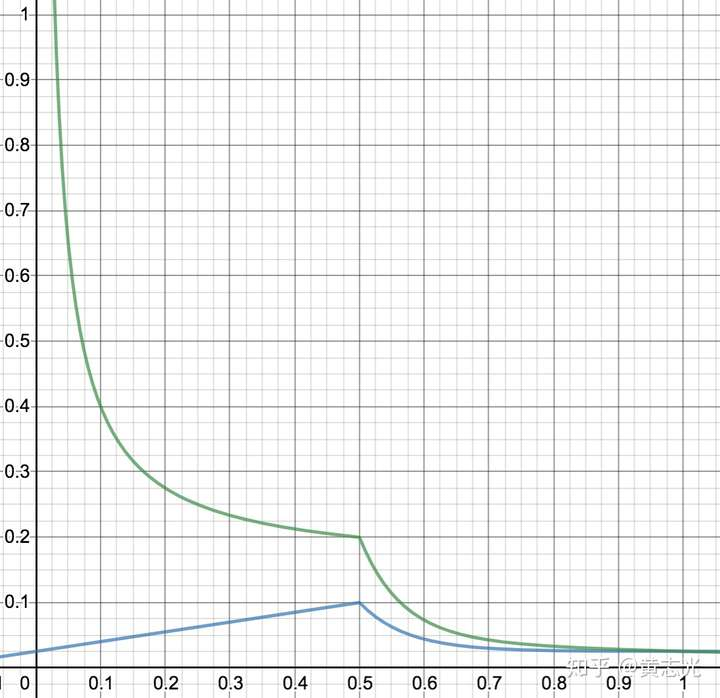DOT的初始发行量是1000万，和其他公链一样，DOT采用通胀模型对验证人和提名人发行新代币用于奖励。不同的是，DOT的通胀数量和通胀率不是固定的，而是通过精妙的算法设计，合理引导代币抵押数量，实现共识安全性和代币流动性。

NPoS的奖励主要来源于DOT代币增发，这也是DOT主要的通胀来源。

1、当抵押率 < 50%，抵押平均年化收益率 > 20%，鼓励更多代币抵押;

2、当抵押率 = 50%，抵押平均年化收益率 = 20%;

3、当抵押率 > 50%，抵押平均年化收益率 < 20%，鼓励赎回而不鼓励抵押。

20%的年化收益率相比传统金融产品具有很大的优势。

### 概念定义

抵押率 x = 抵押DOT的总数 / DOT发行量

年通胀率 R = (年末DOT发行量 - 年初DOT发行量) / 年初DOT发行量

### 定量参数

预期抵押率 x_ideal = 0.5

预期年化收益率i_ideal = 0.2

抵押率为0时的通胀率 R0 = 0.025

衰减率 d = 0.05

### 计算公式

通胀率 R1 = R0 + x * (i_ideal - R0 / x_ideal)

通胀率 R2 = R0 + (i_ideal * x_ideal - R0) * 2 ^ ((x_ideal - x) / d)

通胀率 R = min(R1, R2)

年化收益率 i = R / x

示例数据计算

抵押率 x = 0.4，年化收益率 i = 0.2125，也即 21.25%

抵押率 x = 0.5，年化收益率 i = 0.2，也即 20%

抵押率 x = 0.6，年化收益率 i = 0.0729，也即 7.29%

曲线图《抵押率与通胀率/年化收益率曲线》上图中，横坐标为抵押率，蓝色线纵坐标为年通胀率，绿色线纵坐标为年华收益率。